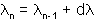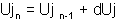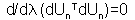# integrator Command

This command is used to construct the Integrator object. The Integrator object determines the meaning of the terms in the system of equation object Ax=B.

The Integrator object is used for the following:

• determine the predictive step for time t+dt
• specify the tangent matrix and residual vector at any iteration
• determine the corrective step based on the displacement increment dU

The system of nonlinear equations is of the form:

 Static analysis:Transient analysis:The type of integrator used in the analysis is dependent on whether it is a or :

 STATIC ANALYSIS* LoadControlDisplacementControlMinUnbalDispNormArcLengthTRANSIENT ANALYSIS Newmark Hilbert-Hughes-Taylor Method (HHT)

static integrators should only be used with a Linear TimeSeries object with a factor of 1.0.